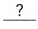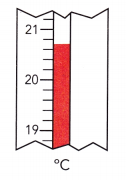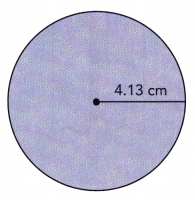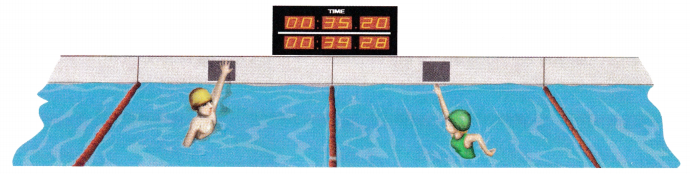# Math in Focus Grade 7 Chapter 1 Lesson 1.5 Answer Key Introducing Significant Digits

Go through the Math in Focus Grade 7 Workbook Answer Key Chapter 1 Lesson 1.5 Introducing Significant Digits to finish your assignments.

## Math in Focus Grade 7 Course 2 A Chapter 1 Lesson 1.5 Answer Key Introducing Significant Digits

### Math in Focus Grade 7 Chapter 1 Lesson 1.5 Guided Practice Answer Key

List the significant digits for each number. Then write the number of significant digits.

Question 1.
23,005
The two zeros are in between nonzero digits. Using RULES 1 and 2,are significant digits.
There aresignificant digits.
There are 3 rules to find the significant figures. They are
Non zero digits are always significant figures.
Any zeros between the two significant figures are also significant.
The zeros in the decimal portion is also significant.
23005 has 5 significant figures.
The 5 significant figures are 2, 3, 0, 0, 5.

Question 2.
367.9410
The trailing zero in the decimal is. Using RULES 1 and 3,are significant digits.
There aresignificant digits.
There are 3 rules to find the significant figures. They are
Non zero digits are always significant figures.
Any zeros between the two significant figures are also significant.
The zeros in the decimal portion is also significant.
All the digits in the 367.9410 is significant.
It has 7 significant figures they are 3, 6, 7, 9, 4, 1, 0.

Question 3.
0.094
There are 3 rules to find the significant figures. They are
Non zero digits are always significant figures.
Any zeros between the two significant figures are also significant.
The zeros in the decimal portion is also significant.
The number 0.094 has 2 significant figures. They are 9 and 4.

Question 4.
450.0
There are 3 rules to find the significant figures. They are
Non zero digits are always significant figures.
Any zeros between the two significant figures are also significant.
The zeros in the decimal portion is also significant.
The number 450.0 has 4 significant figures. They are 4, 5, 0, 0.

Complete.

Question 5.
Round 346 to 2 significant digits.
The third significant digit is 6, which isthan 5.
346 is closer tothan to.
So, the integer rounded to 2 significant digits is.
The third significant digit is 6, which is greater than 5.
346 is closer to 350 than to 340
So, the integer rounded to 2 significant digits is 350

Round each integer to the number of significant digits given.

Question 6.
16,890 (3 significant digits)
In the integer after 3 digits the tens place is greater than 5 so it is written as 16900.
The integer 16890 rounded to the 3 significant digits is 16900.
In the number 16900 the 3 significant numbers are 1,6,9.

Question 7.
96,500,100 (2 significant digits)
In the integer after 2 digits the lakhs place is equal to 5 then it is written has 97000000
The integer 96,500,100 rounded to the 2 significant digits is 97000000.
Tn the number 97000000 The 2 significant digits are 9 and 7.

Question 8.
8,253,611 (4 significant digits)
In the integer after 4 digits the hundred place is greater than 5 then it is written has 8254000
The integer 8253611 rounded to the 4 significant digits is 8254000.
Tn the number 8254000 The 4 significant digits are 8, 2, 5, 4.

Question 9.
7,462 (1 significant digits)
In the integer after 1 digit the hundred place is less than 5 then it is written has 7000
The integer 7462 rounded to the 1 significant digits is 7000.
In the number 7000 The 1 significant digits are 7.

Solve.

The integer 6,590,000 is obtained after rounding a number to the hundreds place.
The integer 200,000 is obtained after rounding another number to the ten thousands place.

Question 10.
List the significant digits in each integer.
There are 3 rules to find the significant figures. They are
Non zero digits are always significant figures.
Any zeros between the two significant figures are also significant.
The zeros in the decimal portion is also significant.
In the number 65,90,000 the significant figures are 6,5,9.
In the number 200000 The significant figure 1s 2.

Question 11.
State the number of significant digits in each integer.
The integer 6,590,000 has 3 significant figures. They are 6,5,9.
The integer 200,00 has 1 significant figures. They are 2.

Complete.

Question 12.
Round 1,230.320 to 5 significant digits.
Using RULE 3, all the digits in 1,230.320 are significant. Only 5 significant digits are required. The sixth significant digit is 2, which isthan 5. So, the decimal rounded to 5 significant digits is.
In the given number after 5 digits from the left the tens place is less than 5 then it is written has 1,230.3
The number 1,230.320 rounded to the 5 significant digits is 1230.3.
In the number 1230.3. The 5 significant digits are 1,2,3,0,3.

Question 13.
Round 0.8765421 to 3 significant digits.
Using RULES 1 and 4, only the digits after the decimal point are significant. Only 3 significant digits are required. The fourth significant digit is 5, which is5. So, the decimal rounded to 3 significant digits is.
In the given number after 3 digits from the left the ten thousands place is greater than 5 then it is written has 0.977
The number 0.8765421 rounded to the 3 significant digits is 0.977.
In the number 0.977. The 5 significant digits are 9,7,7.

Round each decimal to the number of significant digits given.

Question 14.
35.0997 (4 significant digits)
In the given number 35.0997 after 4 digits from the left the tens place is less than 5 then it is written has 35.10
The number 35.997 rounded to the 4 significant digits is 35.10.
In the number 35.997. The 4 significant digits are 3,5,1,0.

Question 15.
0.008010002 (5 significant digits)
In the given number 0.008010002 after 5 digits from the left the ten thousands place is less than 5 then it is written has 0.0080100.
The number 0.008010002 rounded to the 5 significant digits is 0.0080100.
In the number 0.0080100. The 5 significant digits are 0,0,8,0,1.

Question 16.
74.015 (3 significant digits)
In the given number 74.015 after 3 digits from the left the tens place is less than 5 then it is written has 74.0.
The number 74.015 rounded to the 3 significant digits is 74.0.
In the number 74.0. The 3 significant digits are 7,4,0.

Solve.

Question 17.
A reading of a thermometer in Celsius is shown.
a) List the digits in the reading that are certain.
Looking closely at the given thermometer, we found the reading in the thermometer is 20.6°C
The digits that are certain are 2, 0, and 6

b) List the digit that is not certain, the estimated digit. Write an approximate reading with two decimal places.
The reading is in between 20.6° C and 20.7° C
The approximate reading with two decimal places is 20.65°C

c) State the number of significant digits the reading has.There are 4 significant digits in 20.65°C

Solve.

Question 18.
The area of a circle is given by the expression πr2. The radius of a circle is 4.13 centimeters.
a) Calculate the area of the circle.
b) State the area of the circle correct to 3 significant digits.Given that,
The radius of the circle is 4.13 centimeters.
The area of the circle is A = πr2
A = 3.14 x(4.13)2
A = 53.5

### Math in Focus Course 2A Practice 1.5 Answer Key

List the significant digits for each number. Then count the number of significant digits.

Question 1.
0.0017
There are 3 rules to find the significant figures. They are
Non zero digits are always significant figures.
Any zeros between the two significant figures are also significant.
The zeros in the decimal portion is also significant.
The number 0.0017 has 2 significant figures. They are 1 and 7.

Question 2.
82.005
There are 3 rules to find the significant figures. They are
Non zero digits are always significant figures.
Any zeros between the two significant figures are also significant.
The zeros in the decimal portion is also significant.
The number 82.005 has 5 significant figures. They are 8, 2, 0, 0, 5.

Question 3.
300.0
There are 3 rules to find the significant figures. They are
Non zero digits are always significant figures.
Any zeros between the two significant figures are also significant.
The zeros in the decimal portion is also significant.
The number 300.0 has 4 significant figures. They are 3,0,0,0.

Question 4.
0.0600
Rules to Identify Significant Digits in a Given Numbers :
RULE 1 → All nonzero digits are significant.
RULE 2 → Zeros in between nonzero digits are significant.
RULE 3 → Trailing zeros in a decimal are significant.
RULE 4 → Zeros on the left of the first nonzero digit are NOT significant.
RULE 5 → Trailing zeros in any integer may or may not be significant due to rounding.

0.0600

Zeros on the left of the first nonzero digit are not significant,
Using Rule 4, <u> 6, 0 and 0 </u> are significant digits.
There are <u>three</u> significant digits.

6, 0, and 0; Three

Question 5.
45.13
There are 3 rules to find the significant figures. They are
Non-zero digits are always significant figures.
Any zeros between the two significant figures are also significant.
The zeros in the decimal portion are also significant.
The number 45.13 has 4 significant figures. They are 4,5,1,3.

Question 6.
2.002
There are 3 rules to find the significant figures. They are
Non-zero digits are always significant figures.
Any zeros between the two significant figures are also significant.
The zeros in the decimal portion are also significant.
The number 2.002 has 4 significant figures. They are 2,0,0,2.

Round each integer to the number of significant digits stated in the parentheses.

Question 7.
8,496 (to 2 significant digits)
In the given number after 2 digits from the left, the tens place is greater than 5 then it is written as 8500.
The number 8496 rounded to the 2 significant digits is 8500.
In the number 8500, The 2 significant digits are 8,5.

Question 8.
187,204 (to 3 significant digits)
In the given number after 3 digits from the left, the hundred places are less than 5 then it is written has 187000.
The number 187204 rounded to the 3 significant digits is 187000.
In the number 187000. The 3 significant digits are 1,8,7.

Question 9.
39,148 (to 3 significant digits)
In the given number after 3 digits from the left, the tens place is less than 5 then it is written as 39100.
The number 39148 rounded to the 3 significant digits is 39100.
In the number 39100. The 5 significant digits are 3,9,1.

Question 10.
40,100 (to 2 significant digits)
In the given number after 2 digits from the left, the hundred places are less than 5 then it is written as 40000.
The number 40,100 rounded to the 2 significant digits is 40000.
In the number 40000. The 2 significant digits are 4,0.

Question 11.
5,300,924 (to 4 significant digits)
In the given number after 4 digits from the left the hundred places is greater than 5 then it is written as 5301000
The number 5300924 rounded to the 4 significant digits is 5301000.
In the number 5301000. The 4 significant digits are 5,3,0,1.

Question 12.
111,111 (to 4 significant digits)
In the given number after 4 digits from the left, the tens place is less than 5 then it is written as 111100
The number 111,111 rounded to the 4 significant digits is 111100.
In the number 111100. The 4 significant digits are 1,1,1,1.

Question 13.
99,000 (to 3 significant digits)
In the given number after 3 digits from the left, the tens place is less than 5 then it is written as 990
The number 99000 rounded to the 3 significant digits is 990.
In the number 990. The 5 significant digits are 9,9,0.

Question 14.
820,635 (to 1 significant digit)
In the given number after 1 digit from the left, the ten thousand places is less than 5 then it is written as 9000000.
The number 820,635 rounded to the 1 significant digit is 9000000.
In the number 9000000. The 5 significant digits are 9.

Round each decimal to the given number of significant digits.

Question 15.
0.7621 (to 1 significant digit)
In the given number after 1 digits from the left the thousand place is greater than 5 then it is written has 0.8.
The number 0.7621 rounded to the 2 significant digits is 0.8.
In the number 0.8. The 2 significant digits are 8,0.

Question 16.
1 .0087 (to 2 significant digits)
In the given number after 2 digits from the left the hundred place is less than 5 then it is written has 1.0.
The number 1.0087 rounded to the 2 significant digits is 1.0.
In the number 1.0. The 2 significant digits are 1,0.

Question 17.
45.91082 (to 5 significant digits)
In the given number after 5 digits from the left, the tens place is greater than 5 then it is written
45.911.
The number 45.91082 rounded to the 5 significant digits is 45.911.
In the number 45.911. The 5 significant digits are 5,4,9,1,1.

Question 18.
0.08507 (to 3 significant digits)
In the given number after 3 digits from the left, the hundreds place is equal to 5 then it is written as 0.0851.
The number 0.08507 rounded to the 3 significant digits is 0.0851.
In the number 0.0851. The 3 significant digits are 8,5,1.

Question 19.
520.8 (to 3 significant digits)
In the given number after 3 digits from the left the ones place is greater than 5 then it is written has 521.
The number 520.8 rounded to the 3 significant digits is 521.
In the number 521 The 3 significant digits are 5,2,1.

Question 20.
4.381 (to 2 significant digit)
In the given number after 2 digits from the left the tens place is greater than 5 then it is written has 4.4.
The number 4.381 rounded to the 2 significant digits is 4.4.
In the number 4.4. The 2 significant digits are 4,4.

Solve.

Question 21.
Round 0.09845 and 109,530 to the given number of significant digits.
a) 1 significant digit
b) 2 significant digits
c) 3 significant digits
a) 0.09845 rounded to 1 significant digit is 0.10.
109530 rounded to 1 significant digit is 100000.
b) 0.09845 rounded to 2 significant digit is 0.098.
109,530 rounded to 2 significant digit is 0.098.
c) 0.09845 rounded to 3 significant digit is 0.0985
109530 rounded to 3 significant digit is 110000.

Question 22.
The touchpad that Mike hits at the end of a swimming race is calibrated to measure the time to the nearest hundredth of a second. John claims that Mike won the race by 0.005 second. Jacqui says that Mike won by 0.05 second. Whose claim is more reliable? State your reason.John claims : Mike won the race by 0.005 seconds.
Jacqui claims : Mike won the race by 0.05 seconds.
So, 0.05 second is much more reliable than 0.005 seconds.
Hence, Jacqui claim is much more reliable.

Question 23.
Table A measures 2 feet long and table B measures 2.0 feet long.
a) How many significant digits are there in each measurement?
Given that,
The table A measures 2 feet long.
There are 1 significant digit in the 2 feet long.
The table B measures 2.0 feet long.
There are 2 significant figures in the 2.0 feet long.

b) Do you think that the measurements are rounded values? Explain your answer.
If we round Table 2 measurement of 2.0 feet long to 1 significant digit, we see that is rounded to 2 feet long, which is same as Table A measurement.
Thus, the measurement of Table A and Table B are rounded value.
Yes

Question 24.
A bag of potatoes weighs 9.42 pounds on a weighing scale. Which of the significant digits in the scale reading is the least reliable? Explain your answer.
A bag of potatoes weighs 9.42 pounds
The significant digits are 9, 4, and 2
Since, the significant digit 2 is the hundredth reading so, it is least reliable.
2

Question 25.
The thickness of a ream of 500 sheets of paper is 57.15 millimeters. What is the thickness of one sheet of paper correct to 2 significant digits?
Given that,
The thickness of a ream of 500 sheets of paper is 57.15 millimeters.
The thickness of 1 sheet is 500/57.15 is 8.7489.
The correct to the 2 significant digits is 8.7.

Question 26.
Given a rectangle of length 36.80 centimeters and width 13.4 centimeters, find the area of the rectangle correct to 3 significant digits.
Given that,
The length of a rectangle is 36.80 centimeters.
The width of the rectangle is 13.4 centimeters.
The area of the rectangle is length l x B = 36.80 x 13.4 = 13.4 centimeter.
The 13.4 is correct to the 3 significant digit.

Question 27.
The temperature ranges from 43.5°C to 44.5°C in an experiment. You want to find the average of the two extreme temperature readings.
a) What would you write as the value of the average temperature?
Given that,
The temperature range is 43.5°C to 44.5°C.
The average temperature is 44.5°C – 43.5°C = 1°C.

b) How many significant digits will the value of the average temperature have?
In the 1°C the number of significant digits are one they are 1.

Question 28.
The average distance of the Sun from the Earth is about 93,000,000 miles. How many of the trailing zeros could be significant? State your reason.
Given that,
The distance of the sun from from the earth is 9300000 miles.
The trailing zeros means no numbers after continues zero.
The trailing zeros of the number 93000000 is 000000 are 6 zeros.

Brain Work

Use the decimal representation of π, 3.141592653…, to answer the following questions.

a) Describe an irrational number, a, which is at a distance of 0.0001 unit from π.
3.141592653
Irrational number at a distance of 0.0001 unit from π
3.141592653 + 0.0001 = 3.141692653

b) Describe another irrational number, b, which is even closer to π than the previous answer.